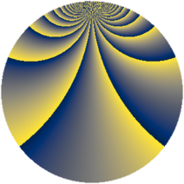# Properties

 Label 2304.3.brLevel $2304$ Weight $3$ Character orbit 2304.br Rep. character $\chi_{2304}(113,\cdot)$ Character field $\Q(\zeta_{48})$ Dimension $3040$ Sturm bound $1152$

# Related objects

## Defining parameters

 Level: $$N$$ $$=$$ $$2304 = 2^{8} \cdot 3^{2}$$ Weight: $$k$$ $$=$$ $$3$$ Character orbit: $$[\chi]$$ $$=$$ 2304.br (of order $$48$$ and degree $$16$$) Character conductor: $$\operatorname{cond}(\chi)$$ $$=$$ $$576$$ Character field: $$\Q(\zeta_{48})$$ Sturm bound: $$1152$$

## Dimensions

The following table gives the dimensions of various subspaces of $$M_{3}(2304, [\chi])$$.

Total New Old
Modular forms 12416 3104 9312
Cusp forms 12160 3040 9120
Eisenstein series 256 64 192

## Trace form

 $$3040q + 16q^{3} - 24q^{5} + 8q^{7} - 16q^{9} + O(q^{10})$$ $$3040q + 16q^{3} - 24q^{5} + 8q^{7} - 16q^{9} + 24q^{11} - 8q^{13} + 16q^{15} + 32q^{19} - 16q^{21} + 24q^{23} - 8q^{25} + 16q^{27} - 24q^{29} - 32q^{37} + 16q^{39} - 24q^{41} + 8q^{43} - 16q^{45} + 24q^{47} - 8q^{49} + 16q^{51} + 32q^{55} - 16q^{57} + 24q^{59} - 8q^{61} + 32q^{63} - 48q^{65} + 8q^{67} - 16q^{69} - 32q^{73} + 16q^{75} - 24q^{77} + 8q^{79} - 16q^{81} + 24q^{83} - 8q^{85} + 16q^{87} + 32q^{91} - 88q^{93} + 16q^{99} + O(q^{100})$$

## Decomposition of $$S_{3}^{\mathrm{new}}(2304, [\chi])$$ into newform subspaces

The newforms in this space have not yet been added to the LMFDB.

## Decomposition of $$S_{3}^{\mathrm{old}}(2304, [\chi])$$ into lower level spaces

$$S_{3}^{\mathrm{old}}(2304, [\chi]) \cong$$ $$S_{3}^{\mathrm{new}}(576, [\chi])$$$$^{\oplus 3}$$